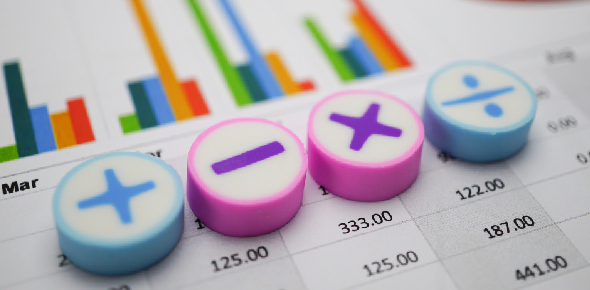# Multiplying And Dividing With Decimals

12 Questions | Total Attempts: 42SettingsThis quiz has decimal questions you need to divide and multiply.

• 1.
2.1 x 5.8 = 12.18
• A.

True

• B.

False

• 2.
12.5 x  18.6
• A.

232.50

• B.

231.95

• C.

101.79

• 3.
48.3 ÷ 8.05 × 9 ÷ 54 × 8.20
• A.

8.2

• B.

1

• C.

9.83

• D.

12.02

• E.

Non o the above

• 4.
0.3 ÷ 8.954
• A.

29.846

• B.

30.78

• C.

Non of the above

• 5.
0.5 ÷ 1.18
• A.

2.86

• B.

2.36

• C.

Non of the above

• 6.
7.8 ÷ 0.3
• A.

26

• B.

2.67

• C.

Non of the above

• 7.
3.6 ÷ 0.18
• A.

23.89

• B.

20

• C.

Non of the above

• 8.
10.6 × 3
• A.

31.8

• B.

34.8

• C.

29.0

• 9.
11.7 × 4.1
• A.

43.96

• B.

47.93

• C.

47.97

• 10.
2.36 × 7.2
• A.

16.992

• B.

14.95

• C.

12.094

• 11.
12.75 × 0.5
• A.

6.095

• B.

6.375

• C.

7.962

• 12.
When multiplying with a decimail you have to line up the decimal.
• A.

True

• B.

False

Related TopicsBack to top